Visitors Online: 0 | Friday 13th December 2019CBSE Guess > Papers > Important Questions > Class XII > 2009 > Maths > Mathematics By Mr. Ashok Khugshal CBSE CLASS XII
Q. 1.Show that f is continuous at x = 1. Q. 2. Differentiate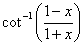w.r.t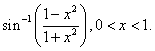Q. 3. Let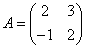and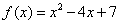. Show that F(A)= 0 .Use this result to find out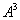. Q. 4. Without expanding show that :-Q. 5. Show that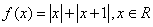is continuous both at x = 0 and x =1. Q. 6. Differentiate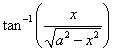with respect to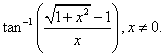Q. 7. Show that. Q. 8. Find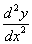if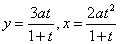Q. 9. Let the two matrices A and B be given byVerify that AB=BA=6I , where I is a unit matrix of order 3 and hence solve the system of equations : x – y =3; 2 x + 3 y +4z =17; Y + 2z = 7 Q. 10. If, findand hence solve the system of equations:Q. 11. Differentiate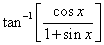with respect to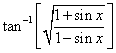. Q. 12. Ifverify that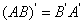. Q. 13. If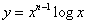, prove that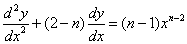Q. 14. Find matrix X for which, where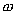is cube root of unity. Q. 15. Find X if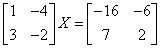Q. 16. Find a and b so that the function f is given byis continuous at x=3 and x=5. Q. 17. If x = sin t y = sin mt , prove that :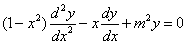Q. 18.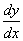when y =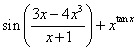Q. 19. Show that the system of equations given below is consistent and hence solve them: Q. 20.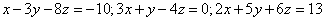Q. 21. If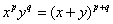, show that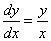, hence show that the result holds good when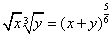. Findwhen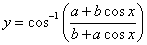Q. 22. Show that :is continuous at x=0. Q. 23. Differentiate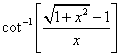w .r. t.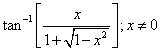Q. 24. If y =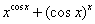, find. Q. 25. Prove that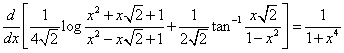. Q. 26. If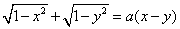then prove that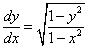Q. 27. If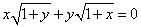; prove that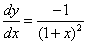Q. 28. For what values of a and b , the following system of equations is consistent?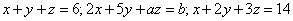. Q. 29. If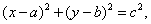Prove thatis a constant independent of a and b. Q. 30. If y =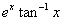than show that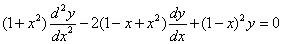Q. 31. Determine the value of ‘k’ so that the function:is continuous at x =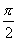Q. 32. If A =, find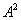and show that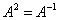. Q. 33. If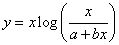, find. Q. 34. If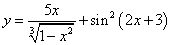, find. Q. 35. If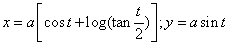thenat t =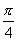. Q. 36. Prove , using properties of determinants thatQ. 37. If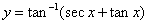find. Q. 38. If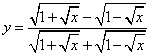, find. Paper By Mr. Ashok Khugshal